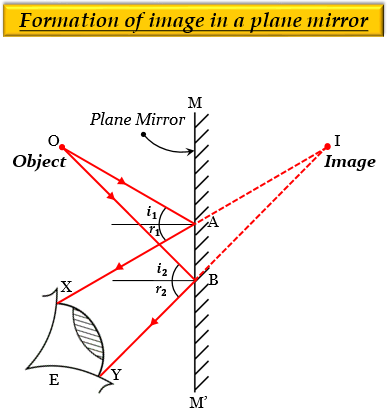# With the help of a labelled ray-diagram, describe how a plane mirror forms an image of a point source of light placed in front of it. State the characteristics of the image formed in a plane mirror.Consider a small object $O$ (a point source of light), placed in front of a plane mirror $MM'$. The mirror will form an image $I$ of the object $O$. This process of image formation is explained as follows:

The object $O$ reflects light rays in all directions. Now, a ray of light $OA$, coming from the object $O$, is incident at point $A$ on the mirror and gets reflected in the direction $AX$ according to the laws of reflection of light, which states that the angle of reflection ${r}_{1}$ equals the angle of incidence ${i}_{1}$. Another ray of light $OB$ coming from the object $O$ strikes the mirror at point B and gets reflected in the direction $BY$, thus, making the angle of reflection ${r}_{2}$ equal to the angle of incidence ${i}_{2}$. The two reflected rays $AX$ and $BY$, are divergent and cannot meet on the left side, so they are produced backwards, where they meet at a point $I$ behind the mirror, which is the image of point source $O$.

Now, when the reflected rays $AX$ and $BY$ enter the eye of a person at position $E$, the eye sees the rays in the direction in which they enter. Therefore, the person looking into the mirror from position $E$ sees the reflected rays as if they are coming from the point $I$ behind the mirror. Thus, the point $I$ is the image of the object $O$ formed by the plane mirror.

Characteristics of the image formed in a plane mirror:

1. The image formed by a plane mirror is virtual (behind the mirror)

2. The image formed by a plane mirror is erect (upright).

3. The size of the image formed by a plane mirror is the same as that of the size of the object.

4. The distance of the image formed by the plane mirror, is equal to the distance of the object from the mirror.

5. The image formed by a plane mirror is laterally inverted, which means the right side of the object appears as the left side of its image and vice-versa (the left side of the object appears as the right side of its image).